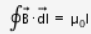# State ampere’s circuital law and express it mathematically

State ampere’s circuital law and express it mathematically.

Ampere’s law states that the path integral or line integral over a closed loop of the magnetic field produced by a current distribution is given byhere I refers to the current enclosed by the loop.
Ampere’s law is a useful relation that is analogous to Gauss’s law of electrostatics. It is a relation between the tangential component of magnetic field at points on a closed curve and the net current through the area bounded by the curve.
To evaluate the expression for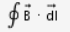, let us consider a long, straight conductor carrying a current I, passing through the centre of a circle of radius r in a plane perpendicular to the conductor.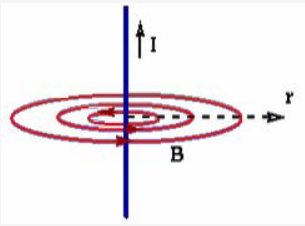According to Biot-Savart law of magnetism, the field has a magnitude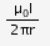at every point on the circle, and it is tangent to the circle at each point.
The line integral ofaround the circle is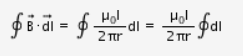Since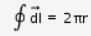is the circumference of the circle,
Therefore,